International
Tables for
Crystallography
Volume B
Reciprocal space
Edited by U. Shmueli

International Tables for Crystallography (2010). Vol. B, ch. 4.6, pp. 600-602   | 1 | 2 |

## Section 4.6.3.1.3. Structure factor

W. Steurera* and T. Haibacha

aLaboratory of Crystallography, Department of Materials, ETH Hönggerberg, HCI G 511, Wolfgang-Pauli-Strasse 10, CH-8093 Zurich, Switzerland
Correspondence e-mail:  walter.steurer@mat.ethz.ch

#### 4.6.3.1.3. Structure factor

| top | pdf |

The structure factor of a periodic structure is defined as the Fourier transform of the density distributionof its unit cell (UC):The same is valid in the case of the (3 + d)D description of IMSs. The parallel- and perpendicular-space components are orthogonal to each other and can be separated. The Fourier transform of the parallel-space component of the electron-density distribution of a single atom gives the usual atomic scattering factors. For the structure-factor calculation, one does not need to useexplicitly. The hyperatoms correspond to the convolution of the electron-density distribution in 3D physical space with the modulation function in dD perpendicular space. Therefore, the Fourier transform of the (3 + d)D hyperatoms is simply the product of the Fourier transformof the physical-space component with the Fourier transform of the perpendicular-space component, the modulation function.

For a general displacive modulation one obtains for the ith coordinateof the kth atom in 3D physical spacewhereare the basic-structure coordinates andare the modulation functions with unit periods in their arguments (Fig. 4.6.3.2). The arguments are, whereare the coordinates of the kth atom referred to the origin of its unit cell andare the phases of the modulation functions. The modulation functionsthemselves can be expressed in terms of a Fourier series as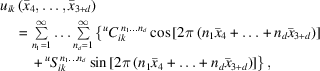whereare the orders of harmonics for the jth modulation wave of the ith component of the kth atom and their amplitudes are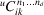and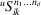.Figure 4.6.3.2 | top | pdf |The relationships between the coordinatesand the modulation functionin a special section of thespace.

Analogous expressions can be derived for a density modulation, i.e., the modulation of the occupation probability: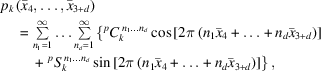and for the modulation of the tensor of thermal parameters: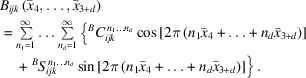The resulting structure-factor formula is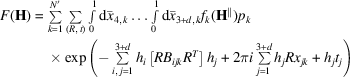for summing over the set (R, t) of superspace symmetry operations and the set of N′ atoms in the asymmetric unit of theunit cell (Yamamoto, 1982). Different approaches without numerical integration based on analytical expressions including Bessel functions have also been developed. For more information see Paciorek & Chapuis (1994), Petricek, Maly & Cisarova (1991), and references therein.

For illustration, some fundamental IMSs will be discussed briefly (see Korekawa, 1967; Böhm, 1977).

Harmonic density modulation. A harmonic density modulation can result on average from an ordered distribution of vacancies on atomic positions. For an IMS with N atoms per unit cell one obtains for a harmonic modulation of the occupancy factor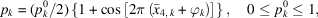the structure-factor formula for the mth order satellite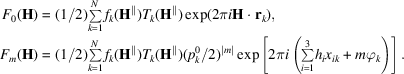Thus, a linear correspondence exists between the structure-factor magnitudes of the satellite reflections and the amplitude of the density modulation. Furthermore, only first-order satellites exist, since the modulation wave consists only of one term. An important criterion for the existence of a density modulation is that a pair of satellites around the origin of the reciprocal lattice exists (Fig. 4.6.3.3).Figure 4.6.3.3 | top | pdf |Schematic diffraction patterns for 3D IMSs with (a) 1D harmonic and (b) rectangular density modulation. The modulation direction is parallel to. In (a) only first-order satellites exist; in (b), all odd-order satellites can be present. In (c), the diffraction pattern of a harmonic displacive modulation alongwith amplitudes parallel tois depicted. Several reflections are indexed. The areas of the circles are proportional to the reflection intensities.

Symmetric rectangular density modulation. The box-function-like modulated occupancy factor can be expanded into a Fourier series,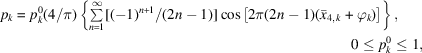and the resulting structure factor of the mth order satellite is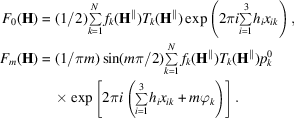According to this formula, only odd-order satellites occur in the diffraction pattern. Their structure-factor magnitudes decrease linearly with the order(Fig. 4.6.3.3b).

Harmonic displacive modulation. The displacement of the atomic coordinates is given by the function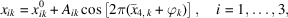and the structure factor by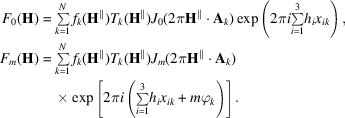The structure-factor magnitudes of the mth-order satellite reflections are a function of the mth-order Bessel functions. The arguments of the Bessel functions are proportional to the scalar products of the amplitude and the diffraction vector. Consequently, the intensity of the satellites will vary characteristically as a function of the length of the diffraction vector. Each main reflection is accompanied by an infinite number of satellite reflections (Figs. 4.6.3.3c and 4.6.3.4).Figure 4.6.3.4 | top | pdf |The relative structure-factor magnitudes of mth-order satellite reflections for a harmonic displacive modulation are proportional to the values of the mth-order Bessel function.

### References

Böhm, H. (1977). Eine erweiterte Theorie der Satellitenreflexe und die Bestimmung der modulierten Struktur des Natriumnitrits. Habilitation thesis, University of Munster.
Korekawa, M. (1967). Theorie der Satellitenreflexe. Habilitation thesis, University of Munich.
Paciorek, W. A. & Chapuis, G. (1994). Generalized Bessel functions in incommensurate structure analysis. Acta Cryst. A50, 194–203.
Petricek, V., Maly, K. & Cisarova, I. (1991). The computing system `JANA'. In Methods of Structural Analysis of Modulated Structures and Quasicrystals, edited by J. M. Pérez-Mato, F. J. Zuniga & G. Madriaga, pp. 262–267. Singapore: World Scientific.
Yamamoto, A. (1982). Structure factor of modulated crystal structures. Acta Cryst. A38, 87–92.